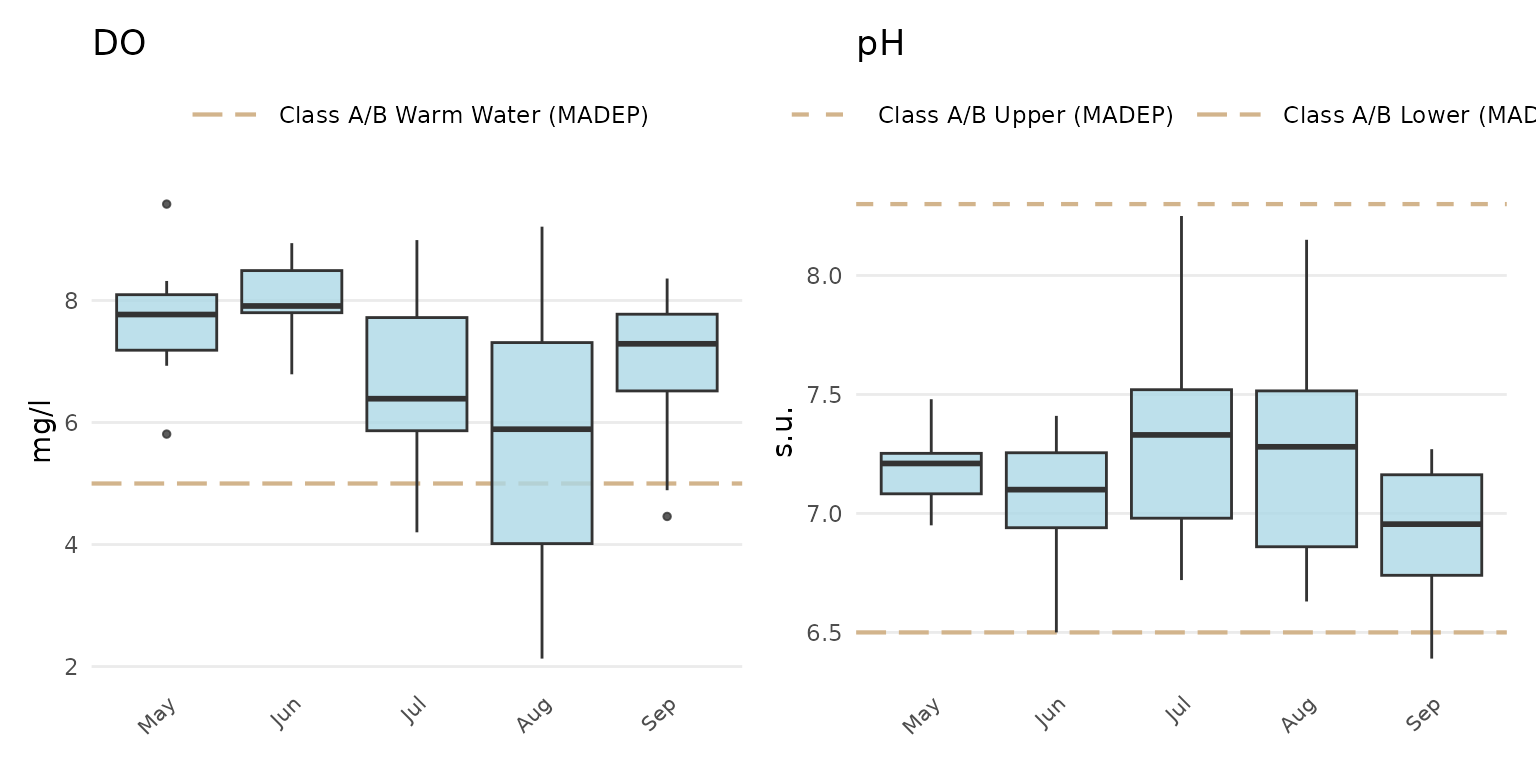The default format for plots created from any of the analyze functions, including the outlier plots, should be sufficient in most cases. However, the plot outputs are ggplot() objects and can be modified using common ggplot() plot functions (see the ggplot2 page for details). A brief overview of ggplot2 is provided here as context to the MassWateR plot modifications below.

## ggplot2

The ggplot2 package was developed following a strict philosophy known as the grammar of graphics. This philosophy was designed to make thinking, reasoning, and communicating about graphs easier by following a few simple rules.

library(ggplot2)

A plot can be created with the function ggplot(). This creates an empty coordinate system for adding layers. The first argument of ggplot() is the dataset to use in the graph. The following creates an empty base graph for the mpg dataset included with ggplot2.

ggplot(data = mpg)

The next step is to add one or more layers (aka geoms) to the ggplot() function. The function geom_point() adds a layer of points to the plot. The ggplot2 package includes many geom functions that each add a different type of layer to a plot. Note the use of the + syntax to build the plot - this is a distinct style of coding that is only used with ggplot2.

ggplot(data = mpg) +
geom_point()

Each geom function in ggplot2 requires a mapping argument. This defines how variables in a dataset are mapped to visual properties. The mapping argument is defined with aes(), and the x and y arguments of aes() specify which variables to map to the x and y axes. The ggplot() function looks for the mapped variable in the data argument, in this case, mpg.

ggplot(data = mpg, mapping = aes(x = displ, y = hwy)) +
geom_point()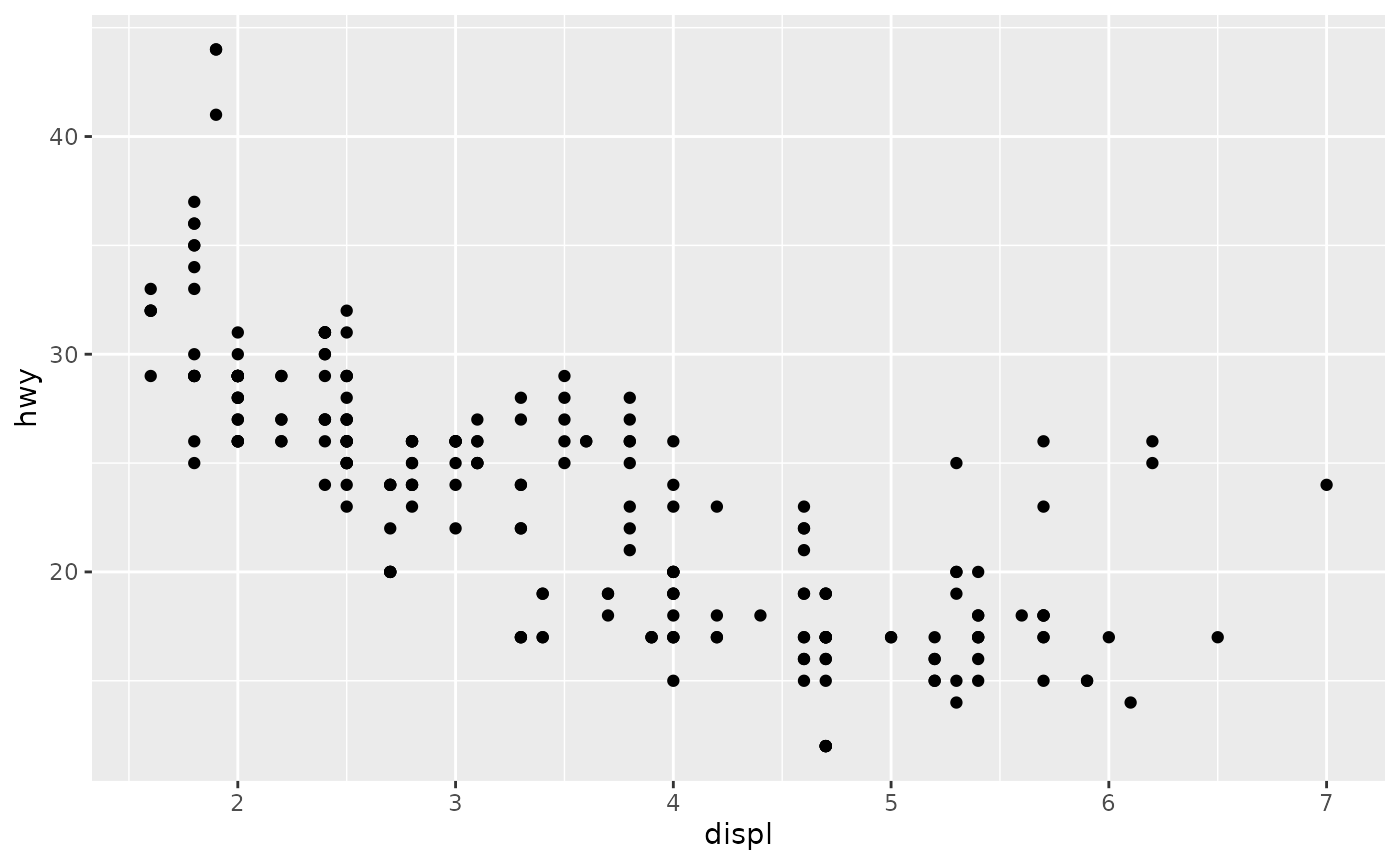Every ggplot follows these rules:

• Each plot starts with the ggplot() function
• Each plot needs three pieces of information: the data, how the data are mapped to the plot aesthetics, and a geom layer

## Modifying MassWateR plots

For plots in MassWateR, additional components can be added using the + notation as for a standard ggplot() object. This is distinct from using any of the default arguments for the plots to change the appearance (e.g., fill = "red"). Overriding some of the existing plot components may also significantly alter the appearance, so use caution. Also note that adding ggplot2 components to an existing MassWateR plot may or may not work based on how the plot is setup. For higher levels of customization, we recommend creating a custom ggplot from scratch, using data from the results file that you loaded for MassWateR. To prepare the results file for use, you can use the utilMWRlimits() function to populate values outside detection limits and remove QC rows.

Before we can modify any plots, the required input files for the MassWateR analyze functions are imported, using the files included with the package for the examples. The checks and warnings are suppressed because we know the files are formatted correctly.

library(MassWateR)

# import results data
respth <- system.file("extdata/ExampleResults.xlsx", package = "MassWateR")
resdat <- readMWRresults(respth, runchk = F, warn = F)

# import accuracy data
accpth <- system.file("extdata/ExampleDQOAccuracy.xlsx", package = "MassWateR")
accdat <- readMWRacc(accpth, runchk = F)

sitpth <- system.file("extdata/ExampleSites.xlsx", package = "MassWateR")
sitdat <- readMWRsites(sitpth, runchk = F)

fsetls <- list(res=resdat, acc=accdat, sit=sitdat)

Below are a few examples of additions to change the standard plot, using anlzMWRseason() for demonstration. A single plot object for the original plot is created as p and is modified differently in each example.

p <- anlzMWRseason(fset = fsetls, param = "DO", thresh = "fresh", group = "month")

Modify plot labels:

p +
labs(
x = "Month",
y = "Dissolved oxygen (mg/L)",
title = "Seasonal summaries for dissolved oxygen"
)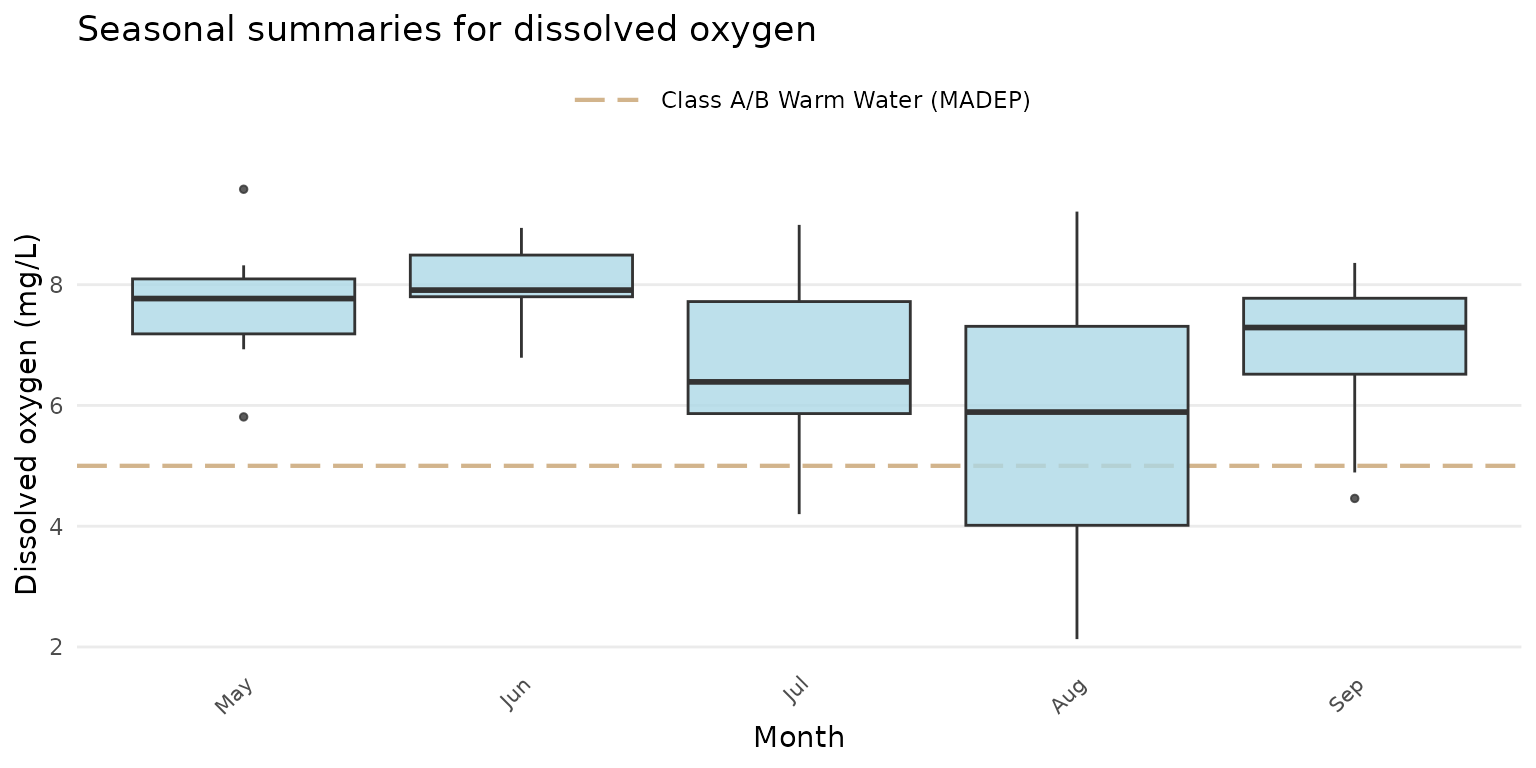Modify the theme:

p + theme_grey()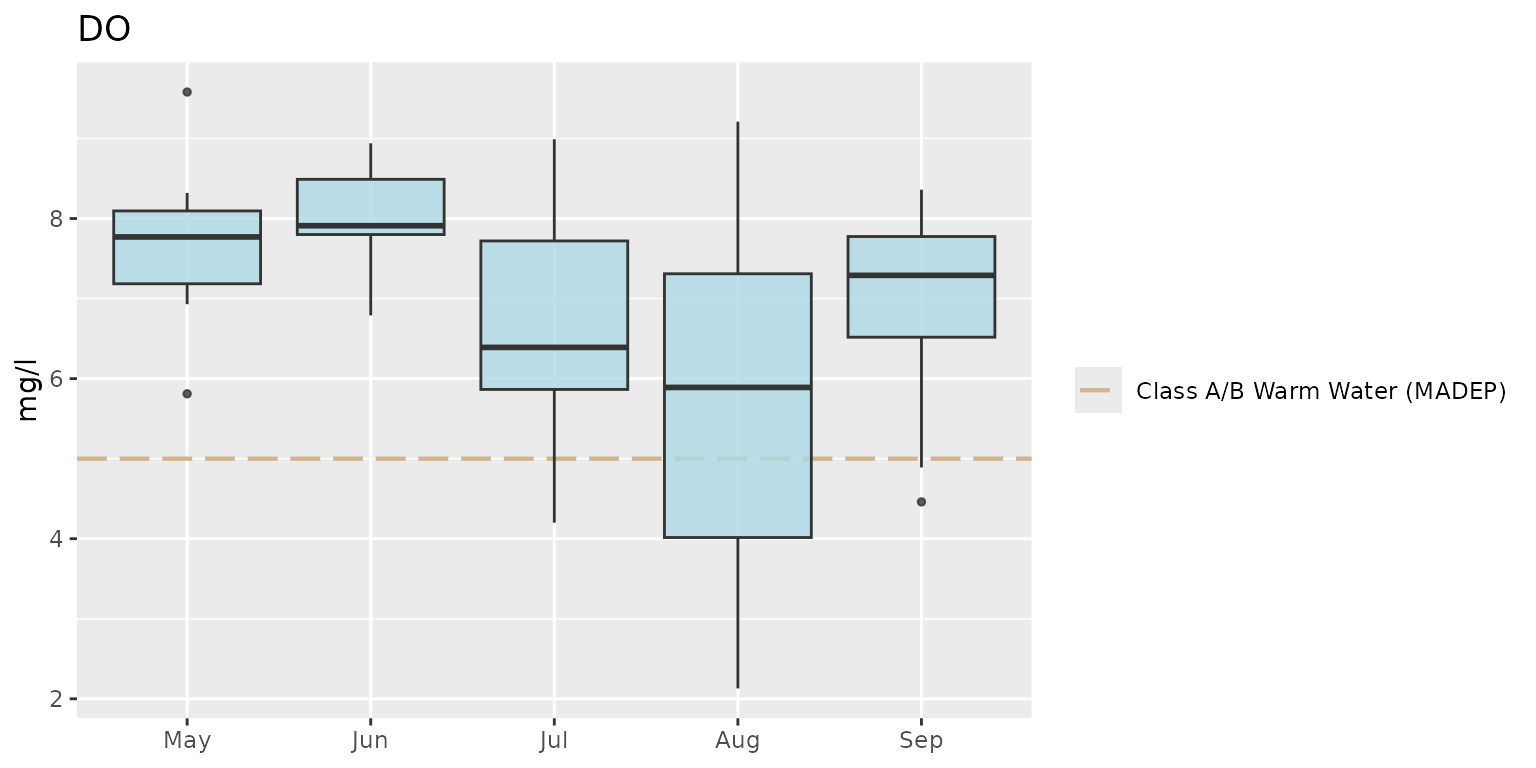Notice how the default legend placement and x-axis text were altered by changing the theme. These can be changed by combining a preset theme (e.g., theme_bw()) with additional theme() elements.

p +
theme_bw() +
theme(
axis.text = element_text(size = 14),
legend.position = "bottom"
)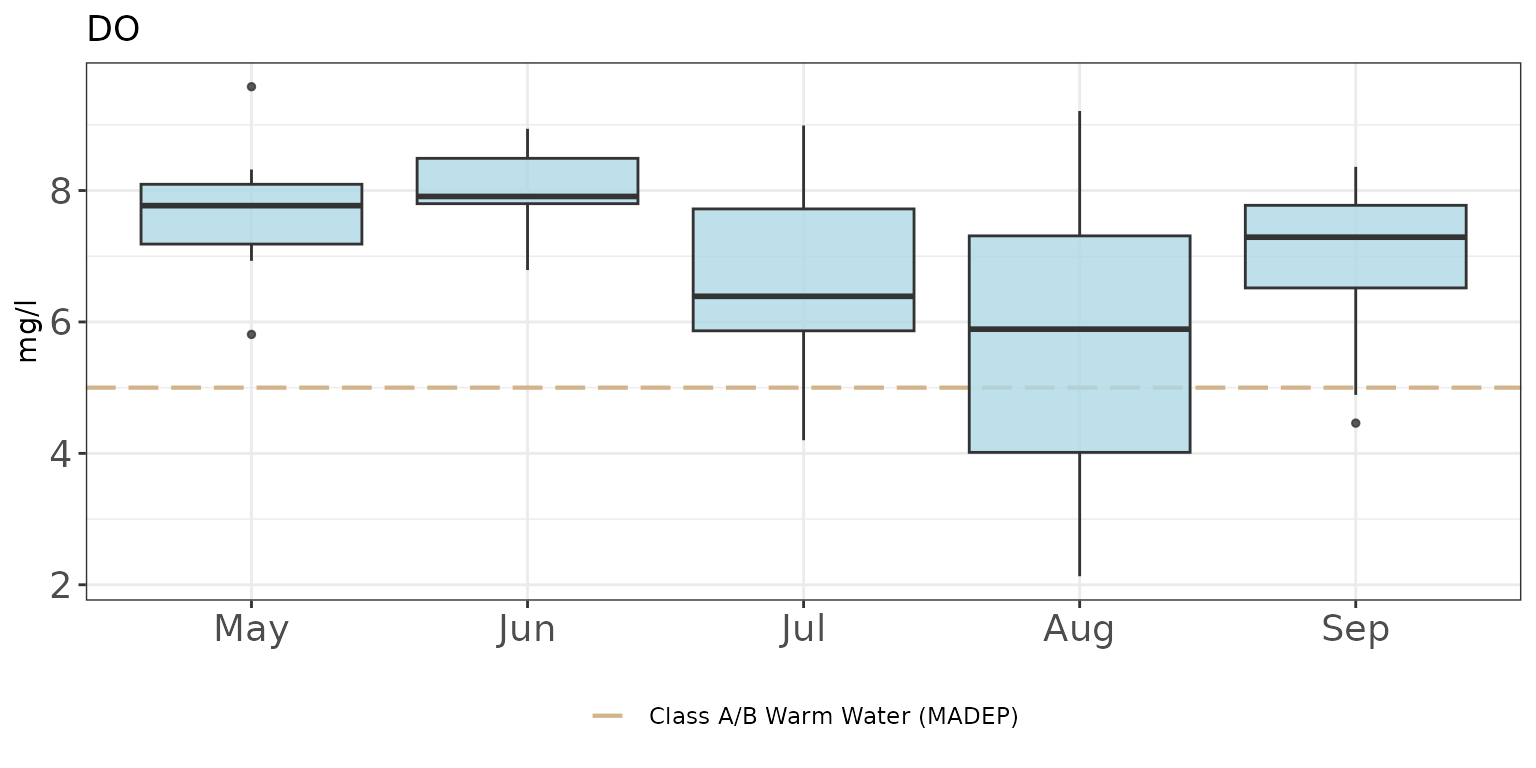The axis limits can be changed using coord_cartesian(). Changing the y-axis requires only the numeric range using the ylim argument.

p + coord_cartesian(ylim = c(0, 8))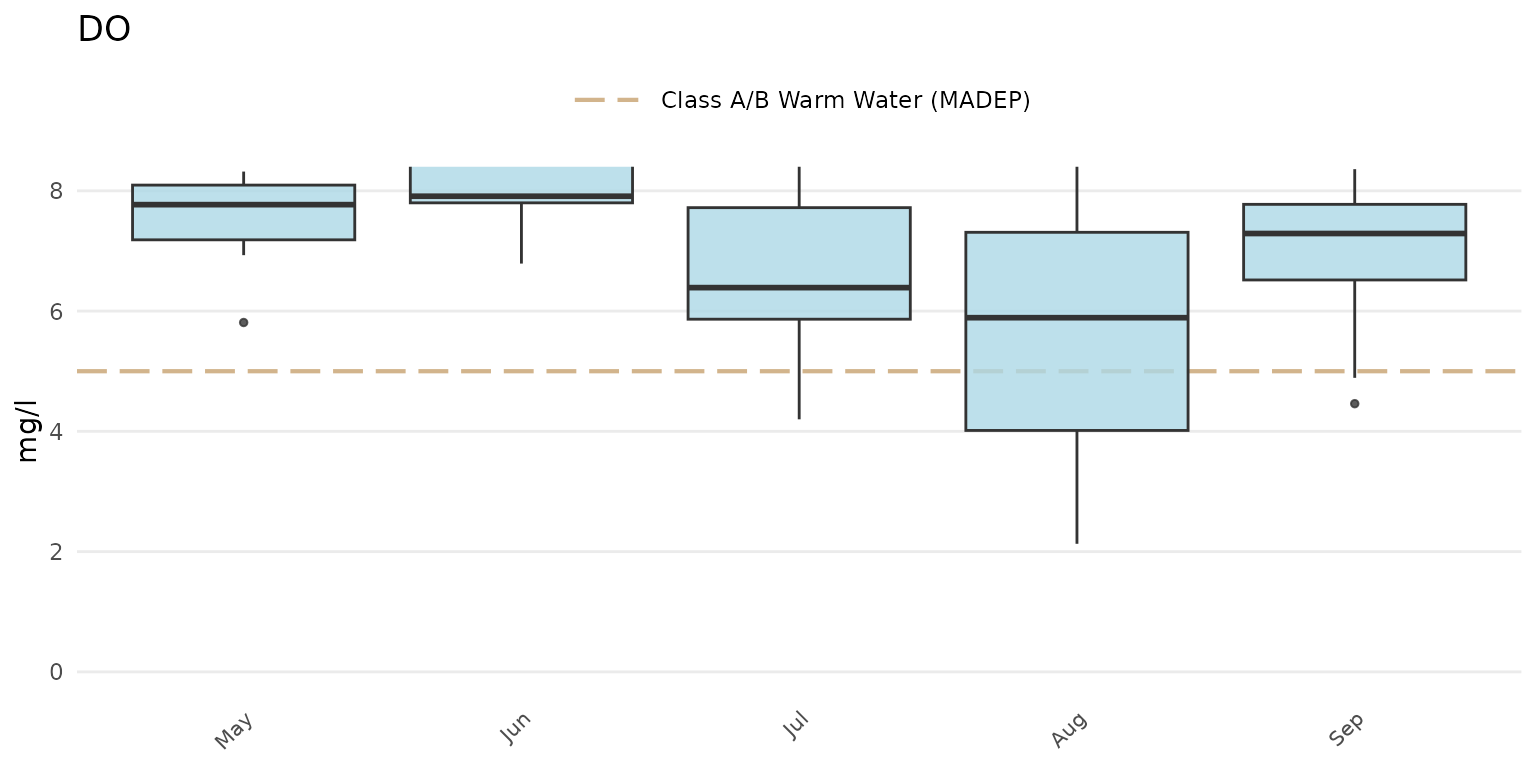A plot with facets for each site:

p + facet_wrap(~Monitoring Location ID)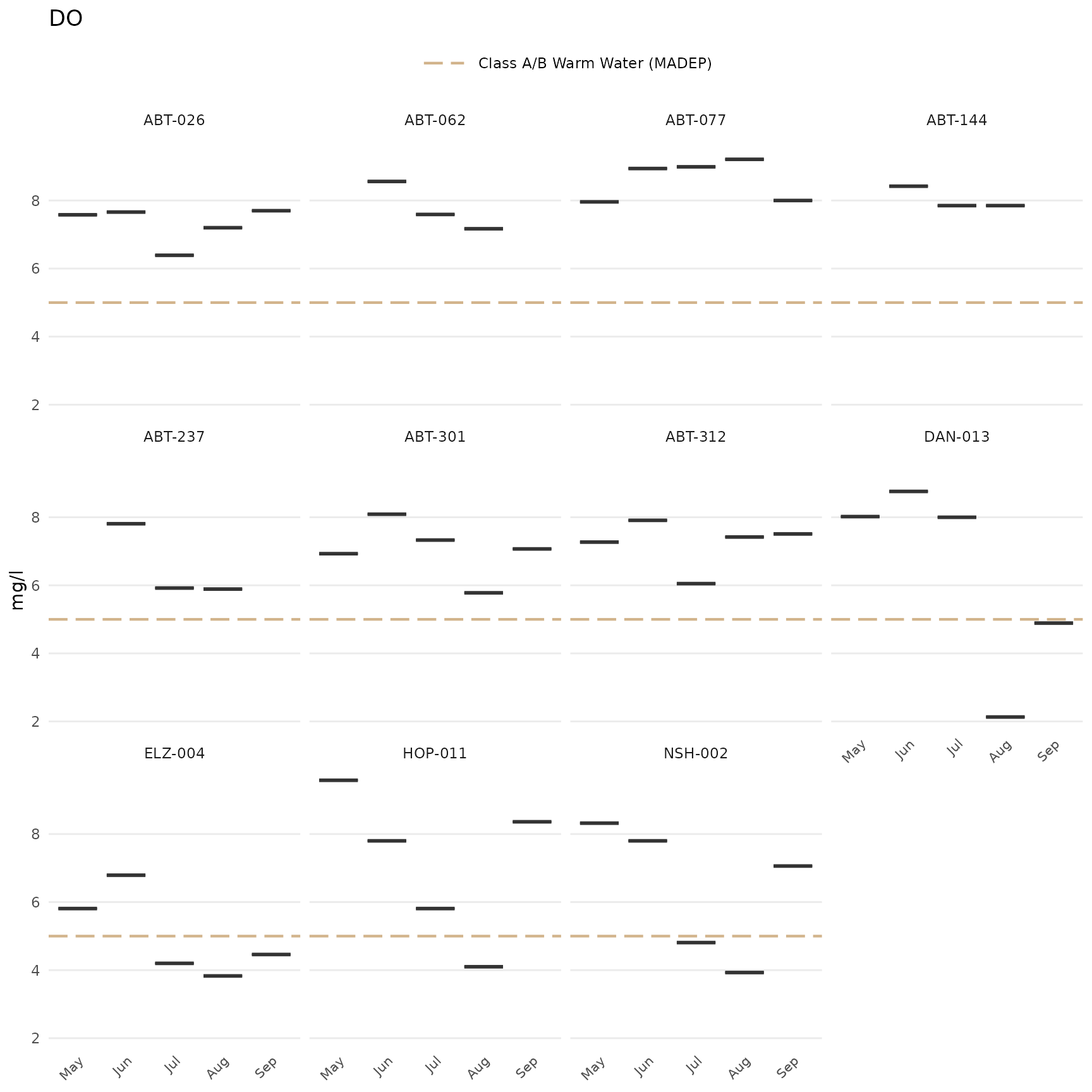Switch the axes:

p + coord_flip()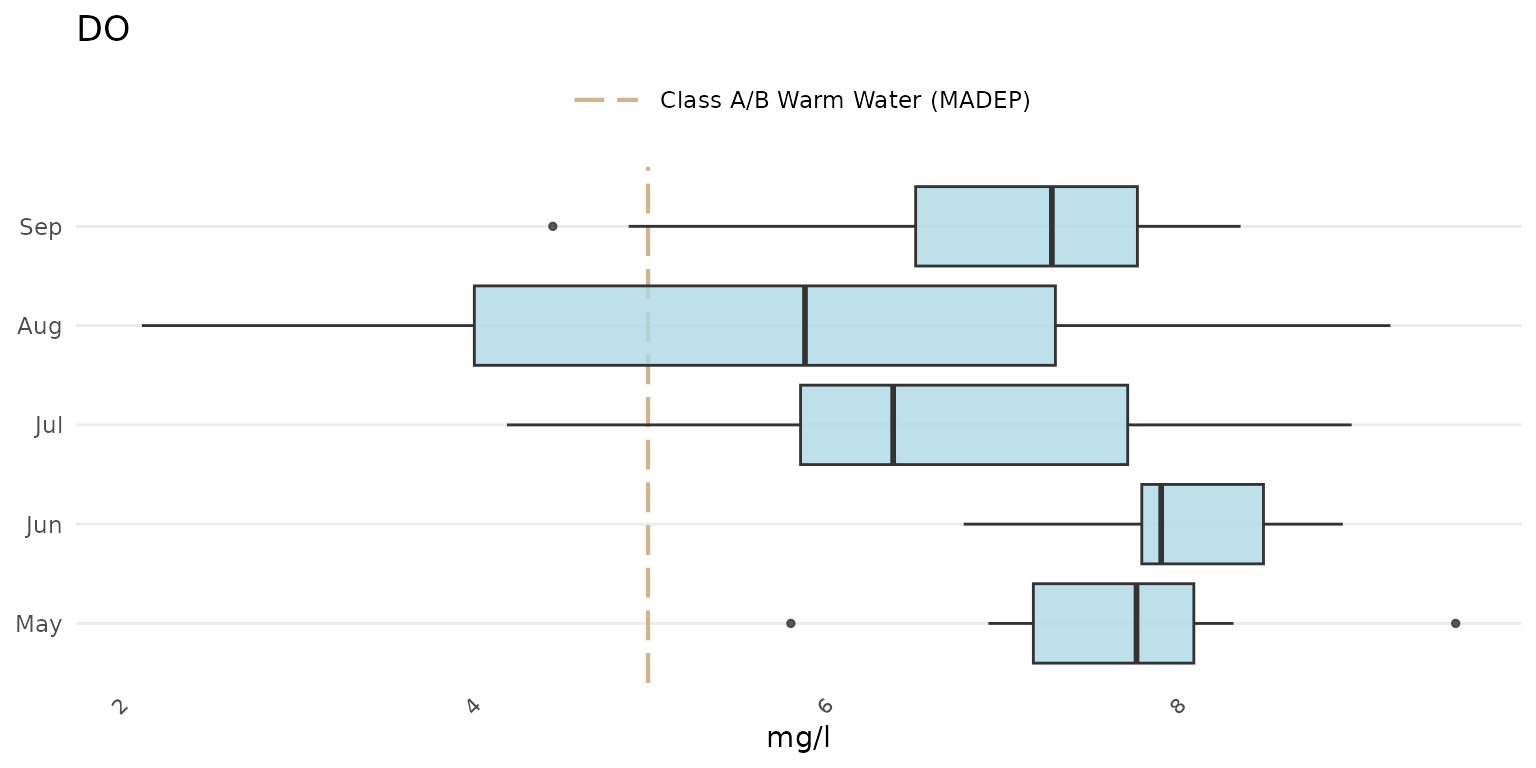Add a custom reference line (although the thresh argument can also be used with a numeric value):

p +
geom_hline(yintercept = 10, linetype = 'solid', color = 'green', size = 2)
#> Warning: Using size aesthetic for lines was deprecated in ggplot2 3.4.0.
#> ℹ Please use linewidth instead.
#> This warning is displayed once every 8 hours.
#> Call lifecycle::last_lifecycle_warnings() to see where this warning was
#> generated.Additional layers can also be added to maps created with anlzMWRmap(). For example, a watershed shapefile can be imported as a simple features object using the sf package, then added using geom_sf() from ggplot. Any warnings about the coordinate system can be ignored. Depending on the watershed boundaries, it may be necessary to adjust the extent of the water features (with buffdist) and/or the boundaries of the map box (with coord_sf()).

library(sf)
library(ggplot2)

# import shapefile as sf object

# use geom_sf to add watershed
anlzMWRmap(fset = fsetls, param = 'DO', addwater = 'high', buffdist = 3) +
geom_sf(data = sudburyMWR) +
coord_sf(xlim = c(-71.68, -71.31), ylim = c(42.20, 42.48))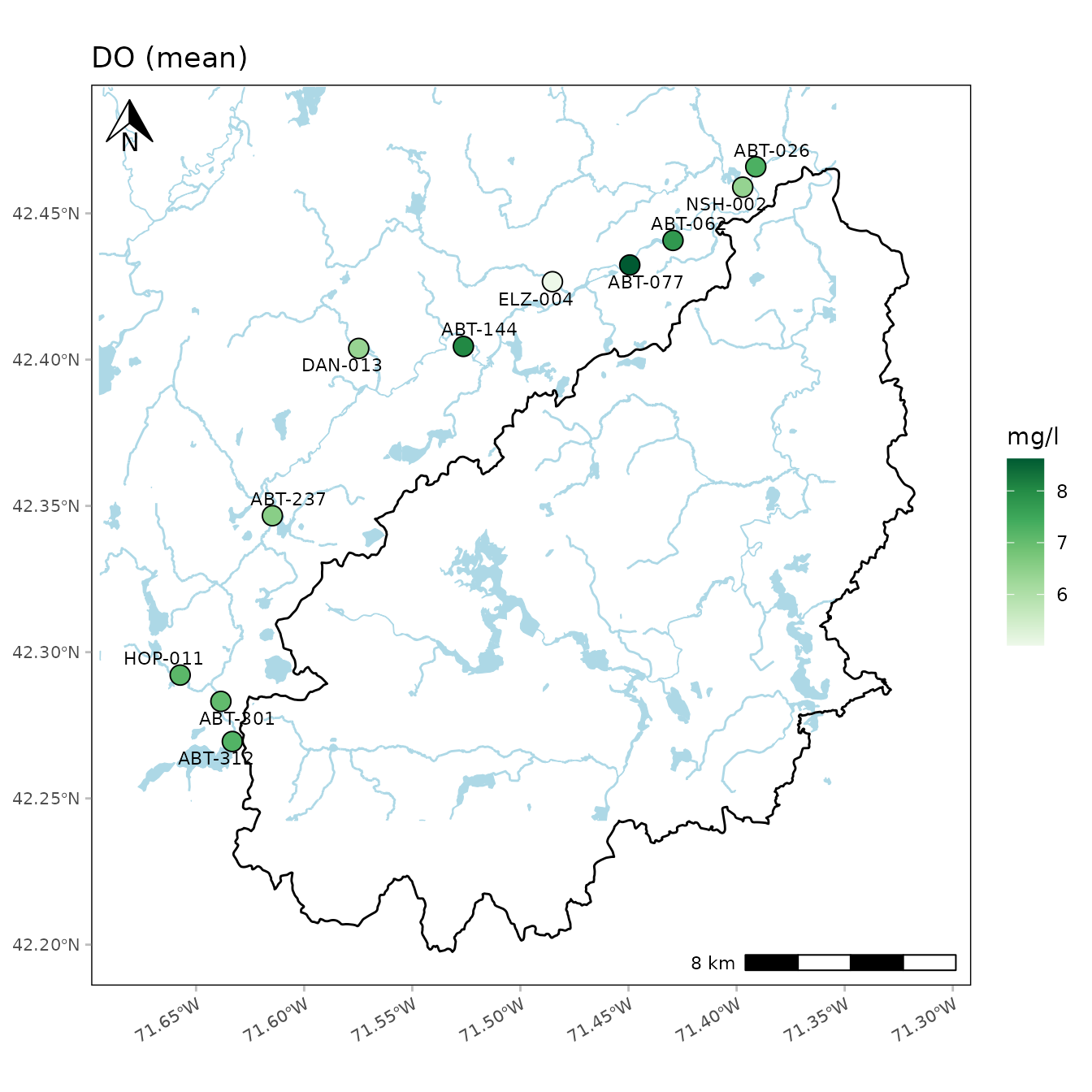Sometimes you might want to place two plots side by side in the same plot window. The patchwork package allows you to easily do this with ggplot. Two or more plots are first created and then combined using the + syntax followed by the plot layout (e.g., plots in two columns using ncol = 2).

library(patchwork)

p1 <- anlzMWRseason(fset = fsetls, param = "DO", thresh = "fresh", group = "month")
p2 <- anlzMWRseason(fset = fsetls, param = "pH", thresh = "fresh", group = "month")

p1 + p2 + plot_layout(ncol = 2)